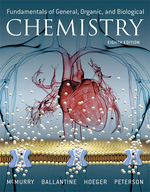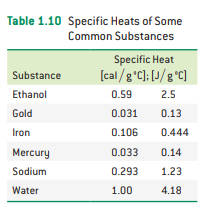×
Get Full Access to Fundamentals Of General, Organic, And Biological Chemistry (Mastering Chemistry) - 8 Edition - Chapter 1 - Problem 1.84
Get Full Access to Fundamentals Of General, Organic, And Biological Chemistry (Mastering Chemistry) - 8 Edition - Chapter 1 - Problem 1.84

×ISBN: 9780134015187 2044

## Solution for problem 1.84 Chapter 1

Fundamentals of General, Organic, and Biological Chemistry (Mastering Chemistry) | 8th Edition

• Textbook Solutions
• 2901 Step-by-step solutions solved by professors and subject experts
• Get 24/7 help from StudySoup virtual teaching assistantsFundamentals of General, Organic, and Biological Chemistry (Mastering Chemistry) | 8th Edition

4 5 1 237 Reviews
25
2
Problem 1.84

A 150 g sample of mercury and a 150 g sample of iron are at an initial temperature of 25.0$${ }^{\circ} \mathrm{C}$$. If 1050 J of heat is applied to each sample, what is the final temperature of each? (See Table 1.10.)Text Transcription:

^circ C

Step-by-Step Solution:
Step 1 of 3

periodicity = rises and falls in trends period = row across group = columns (up & down) atomic radii (size of atom) = across a period, size decreases down a group , size increases (more shells) across a period, # of protons in nucleus "pulls" the e- closer to nucleus An anion (neg) is always larger than its neutral counter part An cation (pos) is always larger than its neutral counter part Paramagnetic = where there is at least one unpaired e- Diamagnetic = all e- are paired .. I.e. Group 1(s2 ﬁlled), noble gases(p6ﬁlled) Metals - tend to make cations (lose electrons) like to oxidize = good reducing agents Non metals - tend to make anions (gains electrons like to reduce = good oxidizing agents Metal oxides (group 1) Basic Nonmetal oxides (co2, h2o, any non metal w oxygen) acidic Amphoteric - can act as an acid or base (semi metals aka metallics , Al2O3, Ge2O3) Strong base = metal will act as acid Strong acid = metal will act as base

Step 2 of 3

Step 3 of 3

##### ISBN: 9780134015187

The full step-by-step solution to problem: 1.84 from chapter: 1 was answered by Aimee Notetaker, our top Chemistry solution expert on 04/25/22, 03:57PM. This textbook survival guide was created for the textbook: Fundamentals of General, Organic, and Biological Chemistry (Mastering Chemistry), edition: 8. This full solution covers the following key subjects: . This expansive textbook survival guide covers 281 chapters, and 240 solutions. Fundamentals of General, Organic, and Biological Chemistry (Mastering Chemistry) was written by Aimee Notetaker and is associated to the ISBN: 9780134015187. Since the solution to 1.84 from 1 chapter was answered, more than 203 students have viewed the full step-by-step answer. The answer to “?A 150 g sample of mercury and a 150 g sample of iron are at an initial temperature of 25.0$${ }^{\circ} \mathrm{C}$$. If 1050 J of heat is applied to each sample, what is the final temperature of each? (See Table 1.10.) Text Transcription:^circ C” is broken down into a number of easy to follow steps, and 45 words.

## Discover and learn what students are asking

Chemistry: The Central Science : Chemical Reactions and Reaction Stoichiometry
?The reaction between reactant A (blue spheres) and reactant B (red spheres) is shown in the following diagram:

Unlock Textbook Solution